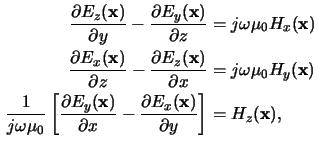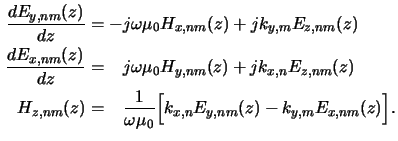Next: 6.2.3 Elimination of the Up: 6.2 Lateral Discretization of Previous: 6.2.1 First Maxwell Equation

## 6.2.2 Second Maxwell Equation

In an exactly analogous way the components of the second Maxwell equation are first written explicitly:and then transformed into the frequency domain. This yields two coupled ODEs for the lateral electric field coefficients Ex, nm(z) and Ey, nm(z) as well as one analytical expression for the vertical magnetic field coefficients Hz, nm(z):Heinrich Kirchauer, Institute for Microelectronics, TU Vienna
1998-04-17